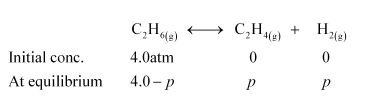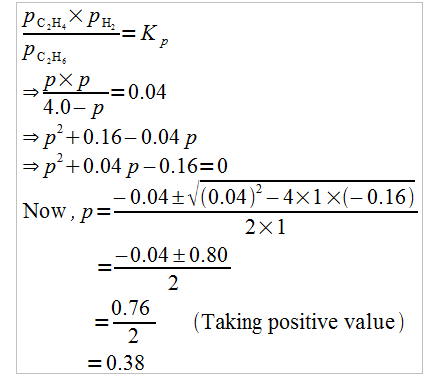# Kp = 0.04 atm at 899 K for the equilibrium shown below`
Question:

$\mathrm{K}_{\mathrm{p}}=0.04 \mathrm{~atm}$ at $899 \mathrm{~K}$ for the equilibrium shown below. What is the equilibrium concentration of $\mathrm{C}_{2} \mathrm{H}_{6}$ when it is placed in a flask at $4.0$ atm pressure and allowed to come to equilibrium?

$\mathrm{C}_{2} \mathrm{H}_{6}(\mathrm{~g}) \longleftrightarrow \mathrm{C}_{2} \mathrm{H}_{4}(\mathrm{~g})+\mathrm{H}_{2}(\mathrm{~g})$

Solution:

Let p be the pressure exerted by ethene and hydrogen gas (each) at equilibrium.

Now, according to the reaction,We can write,Hence, at equilibrium,

$\left[\mathrm{C}_{2} \mathrm{H}_{6}\right]-4-p=4-0.38$

$=3.62 \mathrm{~atm}$